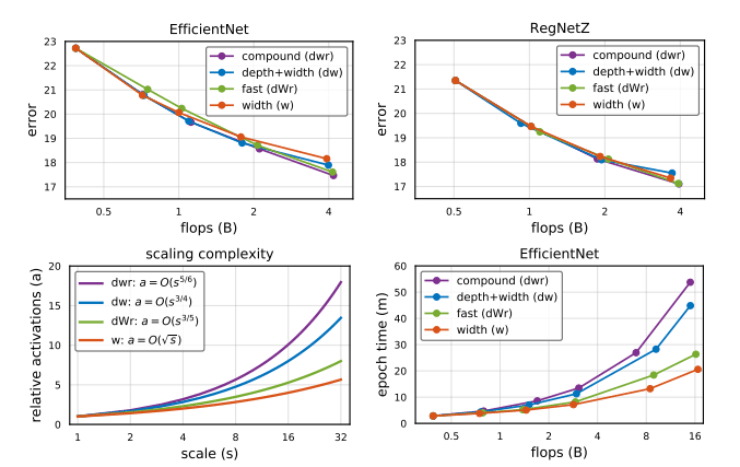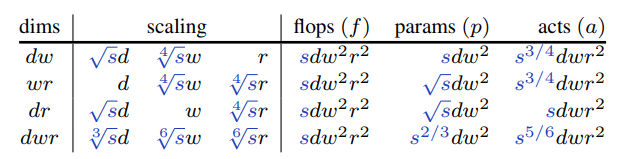# Model Scaling That’s Both Accurate and Fast: Facebook AI Proposes Novel Scaling Analysis Framework and Strategy

A Facebook AI research team explores strategies for convolutional neural network scaling, aiming to provide a framework for analyzing scaling strategies under various computational constraints.

The past several years have seen the rapid development of new hardware for training and running convolutional neural networks. Highly-parallel hardware accelerators such as GPUs and TPUs have enabled machine learning researchers to design and train more complex and accurate neural networks that can be employed in more complex real-life applications.

Regardless of computational resources, it’s necessary to use network scaling methods to build larger models with good accuracy. While existing work on scaling tends to focus on model accuracy, speed is also a key part of network scaling. In a new paper, Facebook AI researchers explore scaling strategies for producing convolutional neural networks that are both fast and accurate; and provide a framework for analyzing such strategies under various computational constraints.

Model scaling is the process of scaling up a base convolutional neural network to endow it with greater computational complexity and consequently more representational power. Model scaling approaches typically focus on maximizing accuracy versus flops performance. The Facebook researchers however note that scaled models with the same flops can have very different runtimes on modern accelerators, and pose the question: Can we design scaling strategies that optimize both accuracy and model runtime?An analysis of four model scaling strategies: width scaling (w), in which only the width of a base model is scaled; compound scaling (dwr), in which the width, depth, and resolution are all scaled in roughly equal proportions; depth and width scaling (dw); and the proposed fast compound scaling (dWr),which emphasizes scaling primarily on, but not only on, model width.

The researchers first apply four different scaling strategies to two base models (EfficientNet-B0 and RegNetZ-500MF) to show that multiple strategies can produce models with similarly high accuracy at the same flops, but that scaling to a fixed target flops using some scaling strategies can result in widely different model runtimes.

To dive deeper into these observed behaviours, the team introduces a general framework for analyzing the relative complexity of various network scaling strategies. The framework uses flops, parameters, and activations as its complexity metrics, where flops represents multiply-add operations, parameters denotes the model’s number of free variables, and activations is the number of elements in the convolutional layers’ output tensors.

With regard to network complexity, the researchers look at convolutional layers, which serve as an excellent proxy of how model scaling affects an entire network. To analyze compound scaling complexity, they scale along multiple dimensions at once.Compound scaling: Complexity of compound scaling strategies with uniform scaling along each dimension, where the relative flops increase of scaling along each dimension is equal.

Because many top-performing networks rely heavily on group and depthwise convolutions, the researchers analyze three basic group convolution scaling strategies (scaling only width, only group, or both), finding that both channel width and group width must be scaled in order to obtain scaling behaviour similar to scaling regular convolutions.Group width scaling: Complexity of scaling a group conv by scaling only width w, only group width g, or both.

After analyzing the behaviour of flops, parameters, and activations for various scaling strategies, the researchers then examine the relationships between these complexity metrics and model runtime.

Given runtime’s strong dependency on activations and the observed properties of different scaling strategies, the researchers introduce their simple and fast compound model scaling idea: design and test scaling strategies that primarily increase model width, but also increase depth and resolution to a lesser extent.

Finally, the researchers conduct experiments on the EfficientNet, RegNetY, and RegNetZ network families to demonstrate how fast scaling can result in both good speed and accuracy.

For EfficientNet, all tested values of α < 1 model accuracy were quite similar and substantially higher than α = 1. For the RegNet baselines, α = 4/5 achieved excellent accuracy and runtime.

The Facebook AI researchers’ framework for analyzing model scaling strategies takes into account not just flops but also parameters and activations, and their proposed fast scaling strategy is shown to result in accurate and fast models. The team hopes the study can provide a general framework for broader reasoning about model scaling.

The paper Fast and Accurate Model Scaling is on arXiv.

Author: Hecate He | Editor: Michael Sarazen

We know you don’t want to miss any news or research breakthroughs. Subscribe to our popular newsletter Synced Global AI Weekly to get weekly AI updates.